Le-Chatelier’s Principle & Factors Affecting Equilibrium Constant (K)

# Le-Chatelier’s Principle & Factors Affecting Equilibrium Constant (K) Notes | Study Physical Chemistry - Chemistry

## Document Description: Le-Chatelier’s Principle & Factors Affecting Equilibrium Constant (K) for Chemistry 2022 is part of Physical Chemistry preparation. The notes and questions for Le-Chatelier’s Principle & Factors Affecting Equilibrium Constant (K) have been prepared according to the Chemistry exam syllabus. Information about Le-Chatelier’s Principle & Factors Affecting Equilibrium Constant (K) covers topics like and Le-Chatelier’s Principle & Factors Affecting Equilibrium Constant (K) Example, for Chemistry 2022 Exam. Find important definitions, questions, notes, meanings, examples, exercises and tests below for Le-Chatelier’s Principle & Factors Affecting Equilibrium Constant (K).

Introduction of Le-Chatelier’s Principle & Factors Affecting Equilibrium Constant (K) in English is available as part of our Physical Chemistry for Chemistry & Le-Chatelier’s Principle & Factors Affecting Equilibrium Constant (K) in Hindi for Physical Chemistry course. Download more important topics related with notes, lectures and mock test series for Chemistry Exam by signing up for free. Chemistry: Le-Chatelier’s Principle & Factors Affecting Equilibrium Constant (K) Notes | Study Physical Chemistry - Chemistry
 1 Crore+ students have signed up on EduRev. Have you?

11. Disturbance in equilibrium: The Le-Chatelier’s Principle

This principle, which is based on the fundamentals of a stable equilibrium, states that “When a chemical reaction at equilibrium is subjected to any stress, then the equilibrium shifts in that direction in which the effect of the stress is reduced”.

Confused with “stress” Well by stress here what we mean is any change of reaction conditions e.g. in temperature, pressure, concentration etc.

This statement will be explained by the following example.

Let us consider the reaction: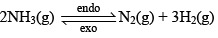Let the moles o f N2, H2 and NH3 at equilibrium be a, b and c moles respectively. Since the reaction is at equilibrium,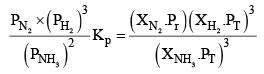Where,

X terms denote respective mole fractions and PT is the total pressure of the system.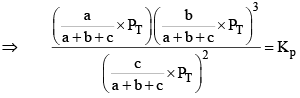Here,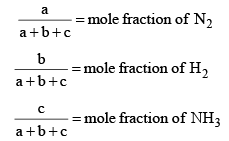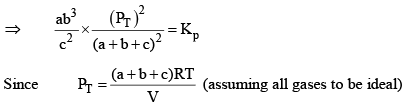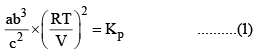Now, let us examine the effect of change in certain parameters such as number of moles. Pressure, temperature etc.

If we increase a or b, the left hand side expressio n becomes QP (as it is disturbed from equilibrium) and we can see that Q> KP

The reaction therefore moves backward to make QP = KP

If we increase c, QP < KP and the reaction has to move forward to revert back to equilibrium.

If we increase the volume of the container (which amounts to decreasing the pressure), QP < KP and the reaction moves forward to attain equilibrium.

If we increase the pressure of the reaction, then equilibrium shifts towards backward direction since in reactant side we have got 2 moles and on product side we have got 4 moles. So pressure is reduced in backward direction.

However from the expression if we increase the temperature of the reaction, the left hand side increase (QP) and therefore does it mean that the reaction goes backward (since QP > KP

Does this also mean that if the number of moles of reactant and product gases are equal no change in the reaction is observed on the changing temperature (as T would not exist on the left hand side)?. The answer to these quest ions is No. This is because KP also change with temperature. Therefore we need to know the effect of temperature on both QP and KP to decide the course of the reaction.

1. Effect of Concentration on Equilibrium:

If the concentration of a component is increased, reaction shifts in a direction which tends to decrease its concentration

For exmaple: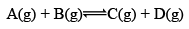At equilibrium: Moles        1        1        1           1          KC = 1

If added 1 mole of D at equilibrium:

1       1          1         1        QC = 2

QC> KC react ion implies reaction proceeds in backward direct ion.

At new equilibrium: 1 +x          1+ x           1-x           2-x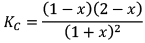Note: Here KC will remain constant even after addition of D Because KC depends on temperature only.

2. Effect of change of pressure / volume on equilibrium

Three cases arises

· Δng = 0

· Δng > 0

· Δng < 0

Case I:Δng = 0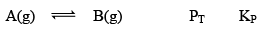At equilibrium 1 atm 1 atm 2 atm       1

When we double the pressure or half the volume at equilibrium at t

2 atm 2 atm 4 atm      1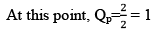Here, K= QP

Hence reaction will not move in any direction. It resembles the reaction is still at equilibrium.

Case II: Δng> 0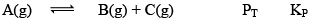At equilibrium  1 atm          1 atm          1 atm             3       1

When we double the pressure or half the volume

At t                     2 atm           2 atm          2 atm            6      2

At this time,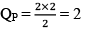QP = 2

QP>KP; hence reaction will move in backward direction.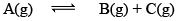At new equation 2+P          2-P          2-P

Case III:Δng < 0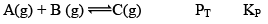At t = teq           1 atm        1 atm          1 atm       3           1

When we double the pressure or half the volume at equilibrium

At t                     2 atm        2 atm       2 atm    6         2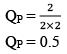Since, QP< KP React ion will move in forward direction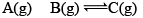New equilibrium      2–P        2–P         2+P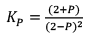Table to calculate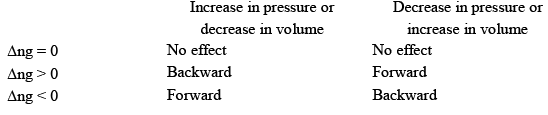3. Effect of Temperature on Equilibrium: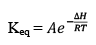Now we will derive the dependence of KP on temperature.

Starting with Arrhenius equation of rate constant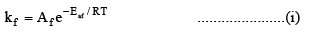Where, k= rate constant for forward reaction, A= Arrhenius constant of forward reaction,

Eaf = Energy of activation of forward reaction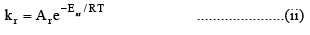Dividing (i) by (ii) we get,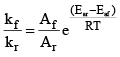We know that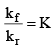(equilibrium constant)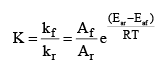At temperature T1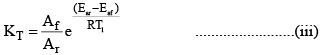At temperature T2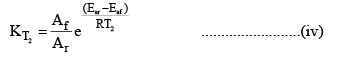Dividing (iv) by (iii) we get

The enthalpy of a reaction is defined in terms of activation energies as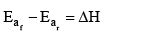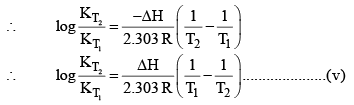For and exothermic reaction, ΔH would be negative. If we increase the temperature of the system (T2 > T1), the right hand side of the equation (V) becomes negative.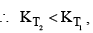that is, the equilibrium constant at the higher temperature would be less than that at the lower temperature.

Now let us analyse our question. Will the reaction go forward or backward?

Before answering this, we must first encounter problem. If temperature is increased. The new KP would either increase or decrease or remain unchanged. If Kp increase and QP decreases.

Then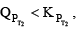therefore the reaction moves forward. If Kincrease and QP remains same then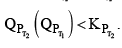Again, the reaction moves forward. What, if KP increase and QP also increase?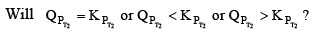This can be answered by simply looking at the dependence of QP and KP on temperature. You can see from the equation that KP depends on temperature exponentially. While Q’s dependence on T would be either to the power g, l, t………..Therefore the variat ion in KP would still be greater QP and the reaction moves forward again. Therefore, to see it decreases, reaction moves backward and if it remains fixed, then no change at all.

Therefore, two cases arises:

(1) ΔH is +ve (Endothermic Reactions)

(2) ΔH is -ve (Exothermic Reactions)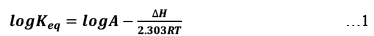Endothermic Reaction: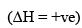As we increase temperature, it is clearly understood by equation (1). That Keq also increase. Hence, with increase in temperature Keq increases in endothermic reactions.

Reactions will shift in forward direction.

Exothermic Reaction (ΔH = -ve)

As temperature is increased, the magnitude o f Keq decreases. Hence, increase in temperature leads to decrease in Keq. React ion will shift in backward direction.

4. Effect of addition of inert gases to a reaction at equilibrium

1. Addition at constant pressure

Let us take a general reaction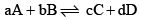We know,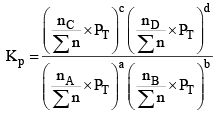Where,

nC , nD , n A , n B denotes the no. of moles of respective components and PT is the total pressure and ∑n = total no. of moles of reactants and products.

Now, rearranging,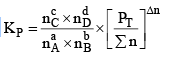Where Δn = (c + d) - (a + b)

Now, Δn can be = 0, < 0 or > 0

Let us take each case separately.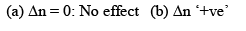Addition of inert gas increases the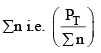is decreased and so is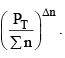So products have to increase and reactants have to decrease to maintain constancy of KP. So the equilibrium moves forward.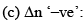In this case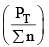decreases but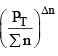increases. So products have to decrease and reactants have to increase to maintain constancy of KP. So the equilibrium moves backward.

2. Addition at Constant Volume:

Since at constant volume, the pressure increases with addition of inert gas and at the same time ∑n also increases, they almost counter balance each other. So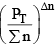can be safely approximated as constant. Thus addition of inert gas has no effect at constant volume.

The document Le-Chatelier’s Principle & Factors Affecting Equilibrium Constant (K) Notes | Study Physical Chemistry - Chemistry is a part of the Chemistry Course Physical Chemistry.
All you need of Chemistry at this link: Chemistry

## Physical Chemistry

67 videos|106 docs|31 tests
 Use Code STAYHOME200 and get INR 200 additional OFF

## Physical Chemistry

67 videos|106 docs|31 tests

Track your progress, build streaks, highlight & save important lessons and more!

,

,

,

,

,

,

,

,

,

,

,

,

,

,

,

,

,

,

,

,

,

;